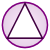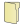Games
Problems
Go Pro!

# Solids

Pro Problems > Math > Geometry > Solids

# Featured Pro ProblemsFind the angle between the diagonals of faces of a cubeFigure out the volume of a wedge of cheese cut from a wheelAn ant wants to traverse an icosahedron along its edges, and have a journey length no more than 4 edges.Calculate the dimensions of the room, given a cubic equation about its volumeAn ant is standing at the top of an icosahedron, and wants to travel to the bottom. How many ways can he do this?Calculate the dimensions of a box, given some information about its volume and dimensionsCalculate the sum of the dimensions of a rectangular prism given its interior diagonal length and surface areaA rectangular prism volume problem involving a cubic equation# Full Directory Listing

Ant on an Icosahedron, Ant on an Icosahedron 2, Cube Diagonals, Warehouse Boxes# Blogs on This SiteReviews and book lists - books we love!The site administrator fields questions from visitors.Like us on Facebook to get updates about new resources